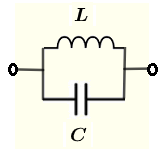# Parallel LC circuit Impedance Calculator

A calculator to calculate the equivalent impedance of an inductor and a capacitor in parallel is presented. Complex numbers in standard form and polar forms are used in the calculations and the presentation of the results.

  

## Formulae for Parallel LC Circuit Impedance Used in Calculator and their UnitsLet $f$ be the frequency, in Hertz, of the source voltage supplying the circuit.
and define the following parameters used in the calculations
$\omega = 2 \pi f$ , angular frequency in rad/s

$X_L = \omega L$ , the inductive reactance in ohms $(\Omega)$
The impedance of the inductor $L$ is given by
$Z_L = j \omega L$

$X_C = 1 / (\omega C)$ , the capacitive reactance in ohms $(\Omega)$
The impedance of the capacitor $C$ is given by
$Z_C = \dfrac{1}{j \omega C} = -\dfrac{j}{\omega C}$

Let $Z$ be the equivalent impedance to the parallel LC circuit shown above and write it in complex form as follows
$\dfrac{1}{Z} = \dfrac{1}{ZL} + \dfrac{1}{ZC}$
which gives
$Z = \dfrac{ZL \; ZC}{ZL + ZC} = \dfrac{(j \omega L)(-\dfrac{j}{\omega C})}{j \omega L-\dfrac{j}{\omega C}} = \dfrac{-j}{\omega C - \dfrac{1}{\omega L}}$

The formulae for the modulus $|Z|$ and argument (or phase) $\theta$ of $Z$ are given by

Modulus: $|Z| = \dfrac{1}{\left| \omega C - \dfrac{1}{\omega L} \right|}$

Argument (Phase): $\theta = - \dfrac{\pi}{2}$ or $- 90^{\circ}$ if $\omega C \gt \dfrac{1}{\omega L}$
Argument (Phase): $\theta = \dfrac{\pi}{2}$ or $90^{\circ}$ if $\omega C \lt \dfrac{1}{\omega L}$
Argument (Phase): $\theta = 0$ if $\omega C = \dfrac{1}{\omega L}$

## Use of the calculator

Enter the resistance, the capacitance and the frequency as positive real numbers with the given units then press "calculate".

 Inductance L = 20 μH mH H Capacitance C = 0.47 pF nF μF mF F Frequency f = 1 GHz MHz kH Hz mHz Number of Decimals 4

## More References and links

AC Circuits Calculators
Complex Numbers - Basic Operations
Complex Numbers in Exponential Form
Complex Numbers in Polar Form
Convert a Complex Number to Polar and Exponential Forms Calculator
Engineering Mathematics with Examples and Solutions